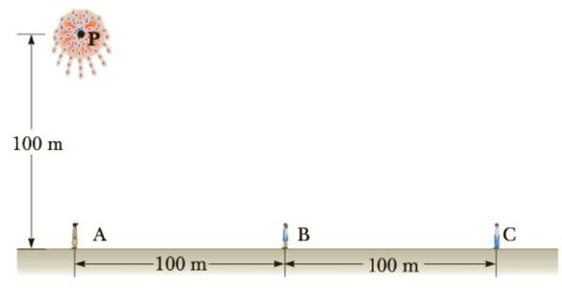Chapter 14, Problem 24P

Chapter
Section
Textbook Problem

A skyrocket explodes 100 m above the ground (Fig. P14.24). Three observers are spaced 100 m apart, with the first (A) directly under the explosion. (a) What is the ratio of the sound intensity heard by observer A to that heard by observer B? (b) What is the ratio of the intensity heard by observer A to that heard by observer C?Figure P14.24

(a)

To determine
The ratio of the sound intensity heard by observer A to that heard by observer B

Explanation

Given Info: distance of the observer A is 100m and distance of the observer B is 100m+100m

Formula to calculate the distance of explosion between P and B is,

rB2=rA2+rAB2

• rB is the distance of the point B from explosion.
• rA is the distance of the point A from explosion.
• rAB is the distance between point A and B.

Formula for ratio of sound intensity of observers

IAIB=rB2rA2

• IA is the sound intensity at observer A.
• IB is the sound intensity at observer B.
• rA is the distance of observer A  from explosion.
• rB is the distance of observer B from explosion.

Use rA2+rAB2 for rB2 to rewrite the above expression

(b)

To determine
The ratio of the sound intensity heard by observer A to that heard by observer C

Still sussing out bartleby?

Check out a sample textbook solution.

See a sample solution

The Solution to Your Study Problems

Bartleby provides explanations to thousands of textbook problems written by our experts, many with advanced degrees!

Get Started

Why does water evaporate?

Chemistry: An Atoms First Approach

Vitamin K is necessary for the synthesis of key bone proteins. T F

Nutrition: Concepts and Controversies - Standalone book (MindTap Course List)

What is the maximum number of bonds that a carbon atom can form?

Biology: The Dynamic Science (MindTap Course List)